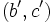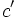# Transposition-preserving automorphism of finitary symmetric group is induced by conjugation by a permutation

## Statement

Let$S$ be a set,$K = \operatorname{Sym}(S)$ be the Symmetric group (?) on$S$, and$G = \operatorname{FSym}(S)$ be the subgroup of$G$ comprising the finitary permutations, i.e.,$H$ is the Finitary symmetric group (?) on$S$. Suppose$\sigma$ is an automorphism of$H$ that sends transpositions to transpositions. Then,$\sigma$ is induced by conjugation by some element of$G$.

## Proof

Given: A finite set$S$.$G$ is the finitary symmetric group on$S$.$\sigma$ is an automorphism of$G$ that sends transpositions to transpositions.

To prove:$\sigma$ is induced by conjugation by a permutation in$K$, the whole symmetric group on$S$.

Proof: By fact (1), it suffices to find$g \in G$ such that conjugation by$g$ agrees with$\sigma$ on the set of transpositions. Further, we can assume that$S$ has at least three elements (The statement is obviously true for$S$ having zero, one, or two elements). We now describe how the permutation$g$ can be constructed explicitly.

Also, we use fact (2) at many steps, without explicitly acknowledging it.

1. Pick distinct elements$a,b,c \in S$. Then, both$\sigma((a,b))$ and$\sigma((a,c))$ are transpositions. We claim that these transpositions have exactly one element in common: If$\sigma((a,b))$ and$\sigma((a,c))$ have no element in common, then they commute, and hence,$(a,b)$ commutes with$(a,c)$, which is not true. Thus,$\sigma((a,b))$ and$\sigma((a,c))$ have exactly one element in commmon.
2. Pick distinct elements$a,b,c \in S$. Then, there are elements$a',b',c' \in S$ such that$\sigma((a,b)) = (a',b')$,$\sigma((b,c)) = (b',c')$ and$\sigma((c,a)) = (c',a')$: This follows directly from the previous step.
3. For any element$a \in S$, there is a unique element$g(a)$ that is involved in the transposition$\sigma((a,b))$ for every$b \ne a$: For any$b \ne c$ distinct from$a$, there exist$a',b',c'$ as described in the previous step. We now show that$\sigma((a,d))$ is also a transposition involving$a'$.
• Since$(a,d)$ commutes with$(b,c)$,$\sigma((a,d))$ commutes with$(b',c')$. In particular, it cannot be a transposition involving$b'$ or$c'$.
• Since$(a,b)$ does not commute with$(a,d)$,$\sigma((a,b))$ does not commute with$\sigma((a,d))$, so they must have an element in common. Thus, either$a'$ or$b'$ is involved in$\sigma((a,d))$.
• These together force$a'$ to be involved in$\sigma((a,d))$.
• We can thus define$g(a)$ as the unique element involved in$\sigma((a,x))$ for every transposition involving$a$.
4. The previous step yields a map$g:S \to S$ such that for any transposition$(a,b)$,$\sigma((a,b))$ is a transposition involving both$g(a)$ and$g(b)$. Step (2) makes it clear that$g(a) \ne g(b)$, so$\sigma((a,b)) = (g(a),g(b))$. Thus,$\sigma$ is induced by conjugation by the permutation$g$.